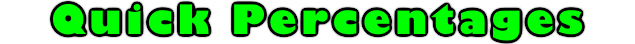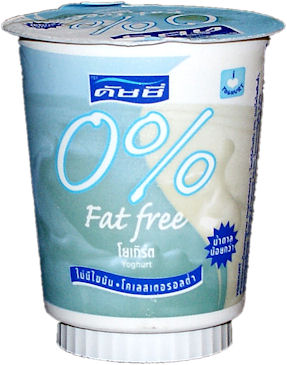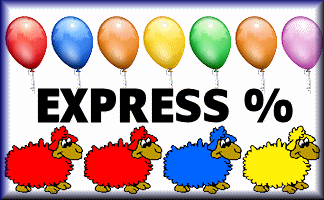### A Maths Starter Of The Day

The answer will be shown here

## Other options:

Topics: Starter | Percentages

• Maggie Harrity, Torquay Devon
•
• Use for KS2 as M/O starter. My year 5 children love it!
• Julie Reakes, The English College, Dubai
•
• It's great to have a starter that's timed and focuses the attention of everyone fully. I told them in advance I would do 10 then record their percentages.
• Mr Bobingson, Sheeepsinvile Primary School
•
• I think this was a jolly good quiz.
• Rachel, Carnoustie High School
•
• This was very good because we have just done percentages and we neded more practice.
• Dawn Roscoe, Auckland
•
• Your other quick calculation exercises have the answers appear after the allotted time which works really well. Any chance of that for this exercise?

[Transum: Thanks for your comments Dawn. A really practical suggestion. As you can see above, an option to show answers has been added.]

How did you use this starter? Can you suggest how teachers could present or develop this resource? Do you have any comments? It is always useful to receive feedback and helps make this free resource even more useful for Maths teachers anywhere in the world.

Previous Day | This starter is for 26 March | Next Day

% - This is the percent symbol.
Percent means 'out of 100'.

### Find 50%:

As 50 is half of 100, then 50% means half. To find 50% of a quantity you need to halve (or divide by two). So 50% of 6 is 3.

### Find 10%:

As 10 is one tenth of 100, then 10% means 'one tenth of'. To find 10% of a quantity you need to divide it by ten. So 10% of 800 is 80.

### Find 25%:

As 25 is one quarter of 100, then 25% means 'one quarter of'. To find 25% of a quantity you need to divide it by four. So 25% of 20 is 5.

Another way of finding 25% of a quantity is first finding 50% then dividing the result by 2.

### Find 33⅓%:

As 33⅓ is one third of 100, then 33⅓% means 'one third of'. To find 33⅓% of a quantity you need to divide it by three. So 33⅓% of 30 is 10.

### Find 1%:

As 1 is one hundredth of 100, then 1% means 'one hundredth of'. To find 1% of a quantity you need to divide it by 100. So 1% of 800 is 8.

### Find other percentages:

Other percentages can be found by combining some of the techniques mentioned above. Here are some examples:

• To find 75% of a quantity add together 50% and 25% of it.
• To find 20% of a quantity double 10% of it.
• To find 5% of a quantity halve 10% of it.
• To find 66⅔% of a quantity double 33⅓% of it.
• To find 90% of a quantity subract 10% from that quantity.
• To find 80% of a quantity subract 20% from that quantity.
• To find 2% of a quantity double 1% of it
• To find 52% of a quantity add 50% to 2% of that quantity

If you need to use a calculator to check your working. See Calculator Workout skill 3.## Other Percentage Starters

Your access to the majority of the Transum resources continues to be free but you can help support the continued growth of the website by doing your Amazon shopping using the links on this page. Below is an Amazon link. As an Amazon Associate I earn a small amount from qualifying purchases which helps pay for the upkeep of this website.

Educational Technology on AmazonTeacher, do your students have access to computers?Do they have iPads or Laptops in Lessons? Whether your students each have a TabletPC, a Surface or a Mac, this activity lends itself to eLearning (Engaged Learning).Transum.org/go/?Start=March26

Here is the URL which will take them to a related student activity.

Transum.org/go/?to=ExpressPercentFor All: